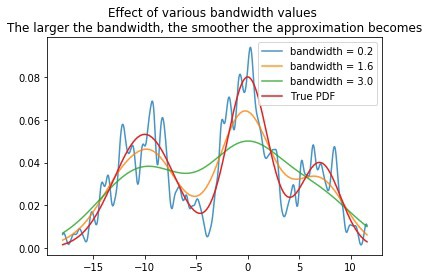# Kernel Density Estimation

## What is a Kernel Density Estimation?

The Kernel Density Estimation is a mathematic process of finding an estimate probability density function of a random variable. The estimation attempts to infer characteristics of a population, based on a finite data set. The data smoothing problem often is used in signal processing and data science, as it is a powerful way to estimate probability density. In short, the technique allows one to create a smooth curve given a set of random data. However, the estimation can also be used to generate points that only appear to have come from a specific sample set. This feature is particularly useful in project simulations and in object modeling.Source

## How does a Kernel Density Estimation work?

The Kernel Density Estimation works by plotting out the data and beginning to create a curve of the distribution. The curve is calculated by weighing the distance of all the points in each specific location along the distribution. If there are more points grouped locally, the estimation is higher as the probability of seeing a point at that location increases. The kernel function is the specific mechanism used to weigh the points across the data set. The bandwidth of the kernel changes its shape. A lower bandwidth limits the scope of the function and leads to the estimate curve looking rough and jagged. By tweaking the parameters of the kernel function (bandwidth and amplitude), one changes the size and shape of the estimate.

### Kernel Density Estimation and Machine Learning

The Kernel Density Estimation technique can be incorporated into machine learning applications. For example, as the estimation function has parameters to define the scope of the kernel, a neural network can begin to train itself to correct its estimations and produce more accurate results. As the estimation process repeats itself, the bandwidth and amplitude estimations are continuously updated to increase the accuracy of the estimated probability density curve.